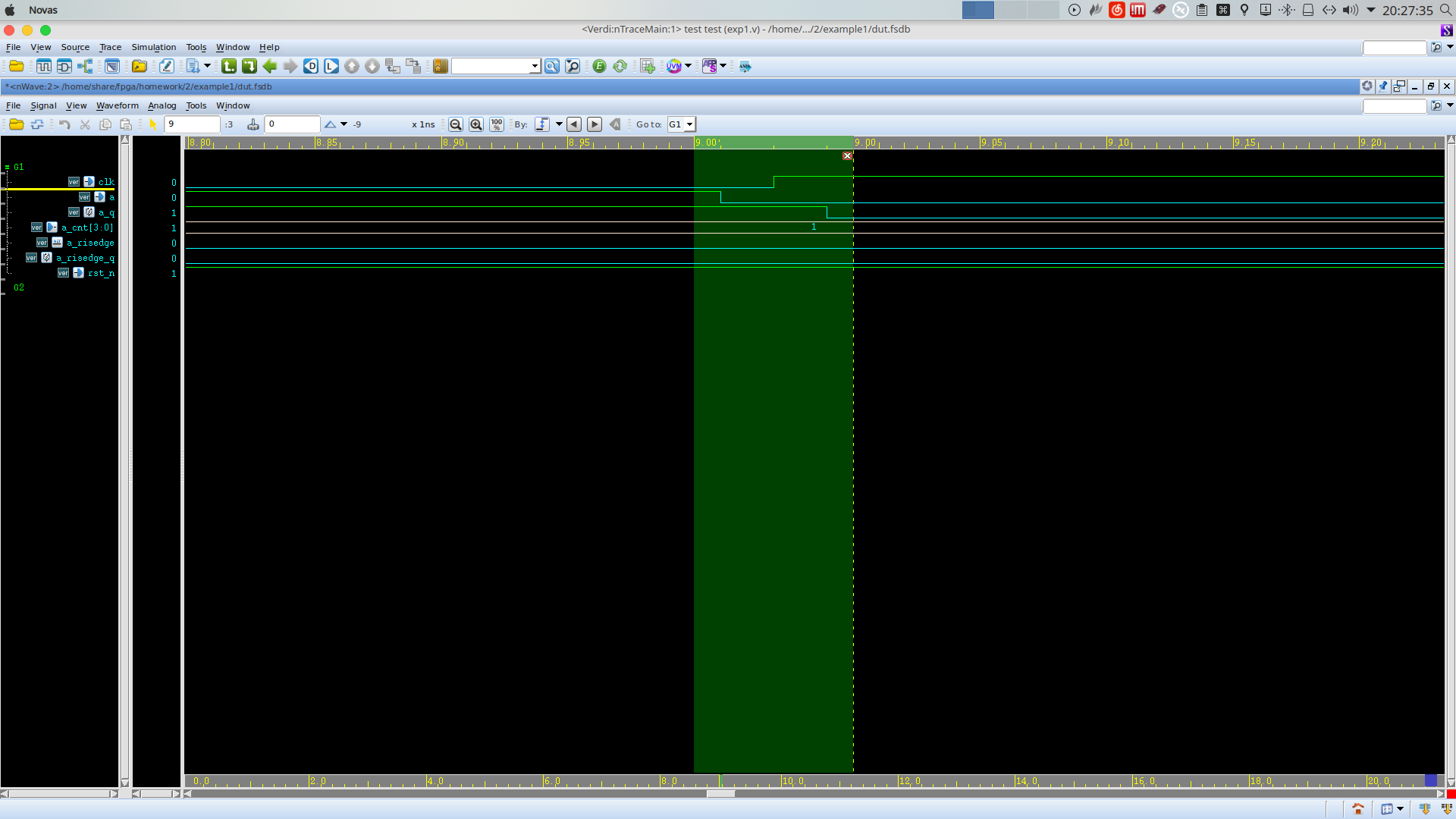# D触发器仿真没有打一拍vcs:2016.06_Full64
verdi: verdi3_L-2016.06-1

```````timescale 1ns/1ps
module exp1(
input        clk   ,
input        rst_n ,
input        a     ,
output [3:0] a_cnt
);
wire a_risedge ;
reg a_risedge_q;
reg a_q        ;
reg [3:0] a_cnt;
assign a_risedge = ( a_q ^ a) & a;
always@(posedge clk or negedge rst_n) begin
if(!rst_n) begin
a_cnt <= 1'b0;
end
else begin
a_q <= a;
end
end
always@(posedge clk or negedge rst_n) begin
if(!rst_n) begin
a_cnt <= 1'b0;
end
else begin
a_risedge_q <= a_risedge;
end
end
always@(posedge clk or negedge rst_n) begin
if(!rst_n) begin
a_cnt <= 1'b0;
end
else if(a_risedge_q == 1'b1)  begin
a_cnt = a_cnt + 1'b1;
end
else begin
a_cnt <= a_cnt;
end
end
endmodule
module test;
wire clk  ;
wire rst_n;
wire a    ;
reg [3:0] a_cnt;
parameter cycle = 2;
exp1 u_exp1(
.clk   (clk  ),
.rst_n (rst_n),
.a     (a    ),
.a_cnt (a_cnt)
);
initial begin
clk = 0;
forever #(cycle/2) clk <= ~clk;
end
initial begin
\$fsdbDumpfile("dut.fsdb");
\$fsdbDumpvars(0,u_exp1);
\$fsdbDumpon();
rst_n = 1'b0;
a 		= 4'b0;
#1;
rst_n = 1'b1;
#1;
a = 1'b1;
#7;
a = 1'b0;
#2;
a = 1'b1;
#4;
a = 1'b0;
#4;
a = 1'b1;
#2;
a = 1'b0;
\$fsdbDumpoff();
\$finish();
end
endmodule``````

``vcs -full64 -cpp g++-4.4 -cc gcc-4.4 -sverilog -fsdb -region exp1.v && ./simv +fsdb+delta``

``verdi -ssf dut.fsdb & ``